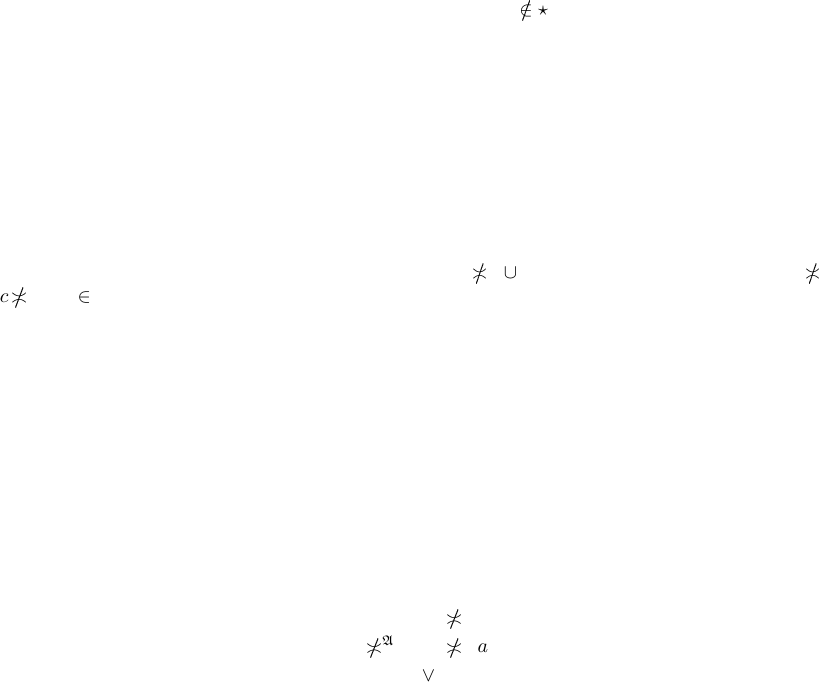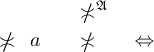Algebraic General Topology. Vol 1: Paperback / E-book || Axiomatic Theory of Formulas: Paperback / E-book

As an Amazon Associate I earn from qualifying purchases.A note on starrish posets
by Victor Porton
78640, Shay Agnon 32-29, Ashkelon, Israel
Email: porton@narod.ru
Web: http://www.mathematics21.org
Abstract
In this note some theorems from my previous article are strengthened. Starrish posets, a
generalization of distributive lattices, are considered.
Keywords: distributive lattice
A.M.S. subject classiﬁcation: 06A06, 06D99
In this short note I strengthen some results about distributive lattices in  as distributive lattices
are a special case of starrish posets introduced in this note.
Deﬁnition 1. I will call a poset starrish when the full star ⋆a is a free st ar for every element a
of this poset.
Proposition 2. Every distributive lattice is starrish.
Proof. Let A is a distributive lattice, a A. Obviously 0
⋆a; obviously ⋆a is an upper set. If
x y a, then (x y) a is non-least that is (x a) (y a) is non-least what is equivalent to
x a or y a being non-least that is x ⋆a y ⋆a.
A generalization of theorem 1 in :
Theorem 3. If A is a starrish join-semilattice then
atoms(a b) = atoms a atoms b
Proof. For every atom c we have: c atoms(a b) c
a b a b ⋆c a ⋆c b ⋆c c
a
c
b c atoms a c atoms b.
A generalization of proposition 30 in :
Proposition 4. Let (A; Z) be a down-aligned ﬁltrator with nitely join-closed core, where A is a
starrish join-semilattice and Z is a join-semilattice. Then atomic elements of this ﬁltrator are prime.
Proof. Let a be an atom of the lattice A. We have fo r every X , Y Z
X
Z
Y up a
X
A
Y up a
X
A
Y a
X
A
Y
A
a
X
A
a Y
A
a
X a Y a
X up a Y up a.
1A generalization of theorem 43 in :
Theorem 5. Let (A; Z) be a star rish join-se milattice ﬁltrator with nitely join-closed core which
is a join-semilattice. Then ∂a is a free s tar for each a A.
Proof. For every A, B Z
X
Z
Y ∂a
X
A
Y ∂a
X
A
Y
A
a
X
A
a Y
A
a
X ∂a Y a.
A generalization of theorem 65 in :
Theorem 6. Let (A; Z) be a semiﬁltered down-aligned ltrator with ﬁnitely meet-closed core Z
which is an atomistic lattice and A is a starrish join-semilattice, then Cor
(a
A
b) = Cor
a
Z
Cor
b
for every a, b A.
Proof. Cor
(a
A
b) =
S
Z
{x | x is an atom of Z, x a
A
b} (use proposition 34 from ),
By the theorem 50 from  we have Cor
(a
A
b) =
S
Z
(atoms
A
(a
A
b) Z) =
S
Z
((atoms
A
a
atoms b) Z) =
S
Z
((atoms
A
a Z) (atoms
A
b Z)) =
S
Z
(atoms
A
a Z)
Z
S
Z
(atoms
A
b Z)
(used the theorem 3). Again using the theorem 50 from , we get
Cor
(a
A
b) =
S
Z
{x | x is an a tom of Z, x a}
Z
S
Z
{x | x is an atom of Z, x b} =
Cor
a
Z
Cor
b (again used the proposition 34 from ).
Bib liography
 Victor Porton. Filters on posets and generalizations. International Journal of Pure and Applied Mathe-
matics, 74(1):55–119, 2012.
2